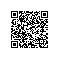# 剑指offer系列之十一：数值的整数次方

package com.rhwayfun.offer;

public class PowerOfDouble {
public double Power(double base, int exponent) {
double result = base;
if(exponent == 0) return 1;
if(exponent > 0){
for (int i = 1; i < exponent; i++) {
result *= base;
}
return result;
}else{
int absExponent = -exponent;
for (int i = 1; i < absExponent; i++) {
result *= base;
}
return 1/result;
}
}

public static void main(String[] args) {
double a = new PowerOfDouble().Power(0.5, -2);
System.out.println(a);
}
}使用钉钉扫一扫加入圈子
+ 订阅